# Compact Lie Group Cheat Sheet

Lie ClassGroup# of generatorsisomorphismsextended Dynkin Diagramdiscrete center
AnSU(n + 1)(n + 1)2 - 1A1 ~ B1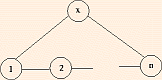Zn + 1
BnSO(2n + 1)n (2n + 1)B1 ~ C1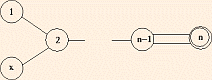CnSp(2n)n (2n + 1)C2 ~ B2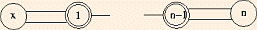Z2
DnSO(2n)n (2n - 1)D2 ~ A1 + A1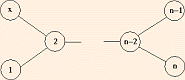Z2
D3 ~ A3
E6E678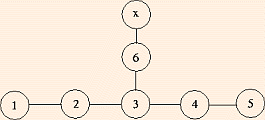E7E7133Z2
E8E8248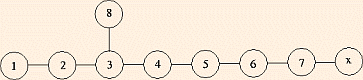Z3
F4F452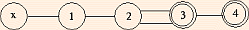G2G214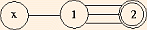Notes:
1. The rank n is the dimension of the Cartan subalgebra (the maximal Abelian subalgebra), whose eigenvalues in the adjoint representation are the roots.
2. SU(n) leaves invariant the quadratic form zi zbari; SO(n) leaves invariant the quadratic form xi xi; Sp(n) leaves invariant the quadratic form in 2n dimensions xi x'n + 1 - i - x'i xn + 1 - i.
3. In the (extended) Dynkin Diagrams, removing a root yields a (maximal) subgroup.